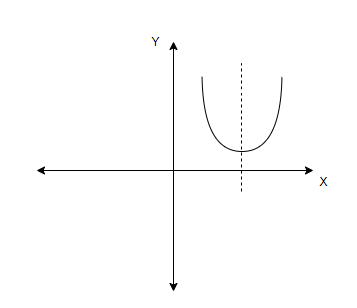Courses
Courses for Kids
Free study material
Free LIVE classes
More# Find the equation of the parabola whose axis is parallel to $y-$axis and which passes through the points $\left( 0,2 \right),\left( -1,0 \right)$ and $\left( 1,6 \right)$.Verified
327.6k+ views
Hint: The general form of the parabola $y=a{{x}^{2}}+bx+c$ is to be used while solving this question.

In the question, it is given that the axis of the parabola is parallel to the $y-$axis. So, the parabola would look like the figure below.We know that the general formula for this form of parabola is given by,
$y=a{{x}^{2}}+bx+c\ldots \ldots \ldots \left( i \right)$
It is given in the question that the parabola passes through the points $\left( 0,2 \right),\left( -1,0 \right)$ and $\left( 1,6 \right)$. Since the parabola passes through the three points, we know that these points must satisfy the equation of the parabola. So, we can substitute each point in the equation $\left( i \right)$ and formulate three sets of equations.
Considering the first point $\left( 0,2 \right)$ and substituting the values of $x=0,y=2$ in equation $\left( i \right)$, we get
\begin{align} & 2=a\times 0+b\times 0+c \\ & c=2\ldots \ldots \ldots \left( ii \right) \\ \end{align}
Considering the second point $\left( -1,0 \right)$ and substituting the values of $x=-1,y=0$ in equation $\left( i \right)$, we get
\begin{align} & 0=a\times {{\left( -1 \right)}^{2}}+b\times -1+c \\ & 0=a-b+c \\ \end{align}
Now substituting the value of $c$ from equation $\left( ii \right)$ in the above equation, we get
\begin{align} & 0=a-b+2 \\ & b-a=2\ldots \ldots \ldots \left( iii \right) \\ \end{align}
Considering the last point $\left( 1,6 \right)$ and substituting the values of $x=1,y=6$ in equation $\left( i \right)$, we get
\begin{align} & 6=a\times {{1}^{2}}+b\times 1+c \\ & 6=a+b+c \\ \end{align}
Now substituting the value of $c$ from equation $\left( ii \right)$ in the above equation, we get
\begin{align} & 6=a+b+2 \\ & a+b=4\ldots \ldots \ldots \left( iv \right) \\ \end{align}
We have two equations $\left( iii \right)$ and $\left( iv \right)$ to get the values of the $a$ and $b$. So, adding the equations,
\dfrac{\begin{align} & b-a=2 \\ & a+b=4 \\ \end{align}}{\begin{align} & 2b=6 \\ & b=3 \\ \end{align}}
Substituting $b=3$ in equation $\left( iii \right)$, we get
\begin{align} & 3-a=2 \\ & a=1 \\ \end{align}
Now we have the values as $a=1,b=3,c=2$. So, we can substitute this in equation $\left( i \right)$,
$y={{x}^{2}}+3x+2$
Therefore, the required equation of the parabola is obtained as $y={{x}^{2}}+3x+2$.

Note: As three points are given in the question, we can formulate three equations and easily compute the three unknowns in the equation. If you are familiar with the cross-multiplication method, you can solve the equations and get the values of $a,b,c$ in less time.
Last updated date: 30th May 2023
Total views: 327.6k
Views today: 6.84k